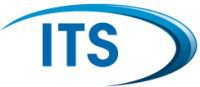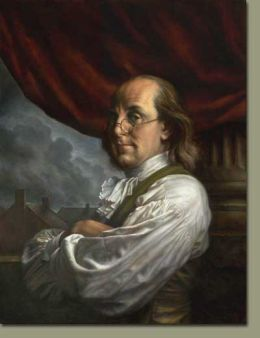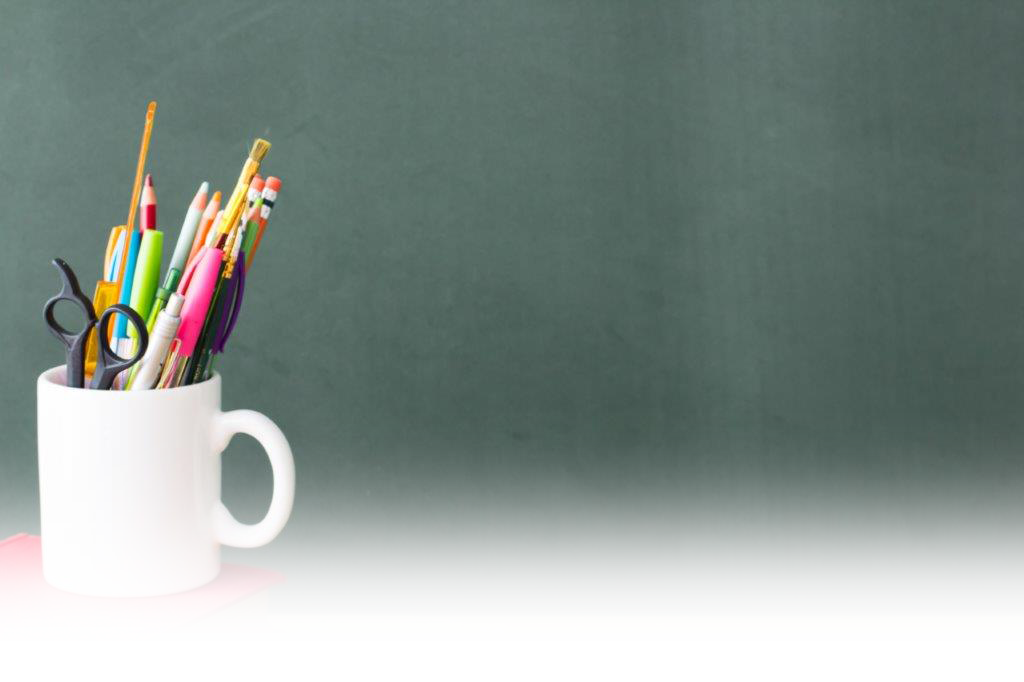Pathways To Learning... Since 2005 Hong Kong Registered School 566985

Menu

# Physics resources for IGCSE or GCSE students

[Science home]

Revision sheets for IGCSE or GCSE physics. This series revision sheets are based on the IGCSE & GCSE syllabuses. Lots of formulas, definitions and key points to learn off. There are also accompanying test sheets to check your memorisation.

The idea is read the information and than print the quiz sheet and try the questions.Benjamin Franklin

Revision Exercise three:

Electricity

Electric charge

Unit of charge: coulomb (C)

metals are electrical conductors

plastic is an example of an insulator

insulating materials can be charged by friction

positive charge à loss of electrons

negative charge à gain of electrons

unlike charges à attract

like charges à repell

eg of use photocopiers and inkjet printers

Mains electricity

hazards of electricity à  frayed cables, long cables, damaged plugs, water around sockets, and pushing metal objects into sockets

current in a resistor à electrical transfer of energy and an increase in temperature

power = current × voltage

× V

Unit of Power: watt (W)

energy transferred = current × voltage × time

× × t

mains electricity is alternating current (a.c.)

direct current (d.c.) à supplied by a cell or battery

Unit of current: ampere (A)

Unit of energy: joule (J)

Unit of resistance: ohm (Ω),

Energy and potential difference in circuits

parallel circuit is more appropriate for domestic lighting

the current in a series circuit depends on the applied voltage and the number and nature of other components

If resistance is reduced the current will increase for a given voltage.

voltage = current × resistance

V = I × R

current is the rate of flow of charge

charge = current × time

Q = I × t

electric current in solid metallic conductors is a flow of negatively

charged electrons

voltage is the energy transferred per unit charge passed

Unit: volt (V) is a joule per coulomb

Quiz for revision exercise three: Electricity quiz

Genius is one percent inspiration and ninety-nine percent perspiration.